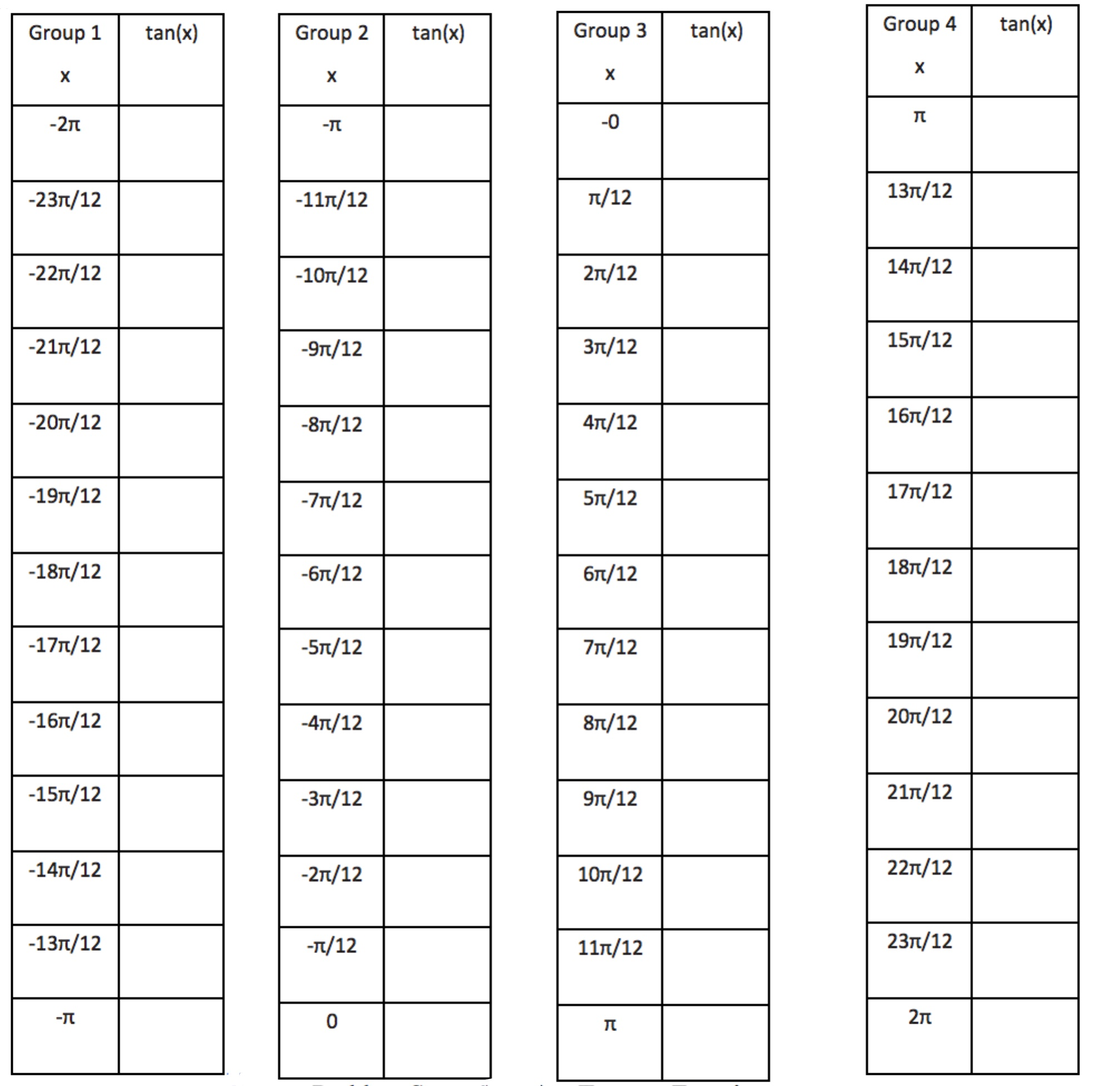# Unit Circle and Trigonometric Functions

## Objective

Graph transformations of tangent functions.

## Common Core Standards

### Core Standards

?

• F.BF.B.3 — Identify the effect on the graph of replacing f(x) by f(x) + k, k f(x), f(kx), and f(x + k) for specific values of k (both positive and negative); find the value of k given the graphs. Experiment with cases and illustrate an explanation of the effects on the graph using technology. Include recognizing even and odd functions from their graphs and algebraic expressions for them.

• F.IF.C.7.E — Graph exponential and logarithmic functions, showing intercepts and end behavior, and trigonometric functions, showing period, midline, and amplitude.

## Criteria for Success

?

1. Identify the period, midline, and vertical asymptotes for the tangent function.
2. Graph simple transformations of the tangent function varying the period, midline, and amplitude.
3. Identify symmetry in the graph of the tangent function.

## Tips for Teachers

?

For the first anchor problem, consider giving each table to a different group and then tiling the graphs together.

## Anchor Problems

?

### Problem 1

Calculate each value of ${\mathrm{tan}(x)}$ to two decimal places for each of the charts below. Then, approximate the graph.#### References

EngageNY Mathematics Algebra II > Module 2 > Topic B > Lesson 14Exploratory Challenge 1

Algebra II > Module 2 > Topic B > Lesson 14 of the New York State Common Core Mathematics Curriculum from EngageNY and Great Minds. © 2015 Great Minds. Licensed by EngageNY of the New York State Education Department under the CC BY-NC-SA 3.0 US license. Accessed Dec. 2, 2016, 5:15 p.m..

Modified by The Match Foundation, Inc.

### Problem 2

For each set of expressions below, all expressions are equivalent. Explain why this makes sense.

${\mathrm{tan}(\pi+x)}$ and ${\mathrm{tan}(2\pi+x)}$

${\mathrm{tan}(-x)}$ and ${\mathrm{tan}(\pi-x)}$ and ${\mathrm{tan}(2\pi-x)}$

#### References

EngageNY Mathematics Algebra II > Module 2 > Topic B > Lesson 14Lesson Summary

Algebra II > Module 2 > Topic B > Lesson 14 of the New York State Common Core Mathematics Curriculum from EngageNY and Great Minds. © 2015 Great Minds. Licensed by EngageNY of the New York State Education Department under the CC BY-NC-SA 3.0 US license. Accessed Dec. 2, 2016, 5:15 p.m..

Modified by The Match Foundation, Inc.

### Problem 3

Graph that function ${f(x)=-2\mathrm{tan}(x)}$.

## Problem Set

?

The following resources include problems and activities aligned to the objective of the lesson that can be used to create your own problem set.

• Also, include some practice with all trigonometric functions focusing on rate of change, increasing, decreasing, maximum, minimum, domain, and range.
• Include problems emphasizing that tangent has a different period than sine and cosine.
• Include problems where students must identify specific points on the tangent function based on reference angles.

a. Sketch a graph of the function ${f(x)=\mathrm{tan}\left(\frac{x}{2}\right)}$.